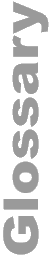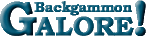Janowski's Takepoint FormulaJanowski's Takepoint Formula A formula devised by Rick Janowski for estimating your take point given your cubeless probability of winning the game. The basic takepoint formula is: ` 2L - 1 TP = ----------- 2W + 2L + x` where TP is the cubeless equity of your take point, L is the average value of your cubeless losses (e.g., −1, if you can't lose a gammon), W is the average value of your cubeless wins (e.g., +1 if you can't win a gammon), and x is a number between 0 and 1 (typically 0.55 to 0.8) that measures cube efficiency. See Janowski's article, Take-Points in Money Games.

 Help   •   About the Glossary   •   List of Terms   •   Full Glossary